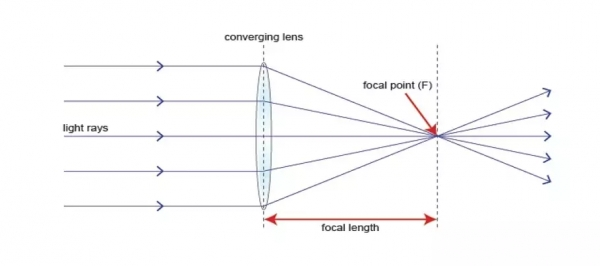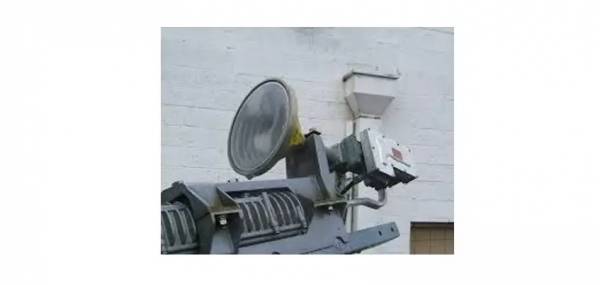# How does a Lens antenna work?

The lens antenna uses a curved surface for the transmission or reception. Lens antennas are made up of glass, where the converging and diverging properties of the lens are followed. These are used for higher frequency applications greater at 3000 MHz and above.

## Working Principle

• If a light source is assumed to be there at a focal point of a lens, which is at a focal distance from the lens, then the rays get through the lens as collimated or parallel rays on the plane wavefront.

• The rays that pass through the center of the lens are less refracted than the rays that pass through the edges of the lens. All of the rays are sent to be in parallel to the plane wavefront. This phenomenon of the lens is called as divergence.

• The same procedure gets reversed if a light beam is sent from right side to left of the same lens. Then the beam gets refracted and meets at a point called focal point, at a focal distance from the lens. This phenomenon is called convergence.The above image represents the focal point and focal length from the source to the lens. The parallel rays obtained are also called the collimated rays. In the above figure, the source at the focal point, at a focal distance from the lens, gets collimated in the plane wavefront. This phenomenon can be reversed which means the light is sent from the left side, gets converged at the right side of the lens.

It is because of this reciprocity, the lens can be used as an antenna, as the same phenomenon helps in utilizing the same antenna for both transmission and reception.The above shown is the image of the model of a lens antenna.

To achieve the focusing properties at higher frequencies, the refractive index should be less than unity. So whatever may be the refractive index, the purpose of the lens is to straighten out the waveform. Based on this the E-plane and H-plane lens are developed, which also delay or speed up the wavefront. This is how a lens antenna works.